JEE## Introduction to Energetics or Chemical Energetics

Let's see what happens if we pour a drop of water into an uncovered petri dish. The water evaporates after a few minutes. Let's try a different experiment in a covered petri dish. What is going to happen? After the same time interval as the first experiment, it remains on the plate.

We can ask a number of questions about these experiments. What causes these events? How quickly do they occur? Is it possible to provide a chemical explanation for these events? What is the name of this procedure? What is the name of the reversal procedure? Where have you seen it in your day-to-day life? What other effects did the initial process have? Thermochemistry, kinetics, and thermodynamics are used to study all these topics. As a result, chemical energetics plays a significant role in advanced physical chemistry.

### Important Topics for Chemical Energetics

• Enthalpy Change

• Hess Law

• Born - Haber Cycle

• Bond Energies

• Reaction Pathway

• Entropy Change

• Gibbs Free Energy

• Spontaneity

• Glycolysis

### Enthalpy change, ΔH

• The thermal energy held in a chemical system is known as enthalpy. It can't be directly measured.

• Energy changes occur as a result of chemical processes (typically heat energy changes).

• The reaction is exothermic when energy is discharged into the environment, and H is negative. The reaction is endothermic and H is positive if energy is taken in from the environment.

• During an exothermic reaction, the temperature of the environment rises, while during an endothermic reaction, it falls.

• The energy required to break one mole of a particular gaseous bond and produce atoms is referred to as bond energy.

• As the actual enthalpy of a bond changes depending on which molecule it is in, bond enthalpies are given as a mean average in the data book.

• Breaking bonds requires energy, hence ΔH is positive (endothermic). When bonds are formed, energy is released, hence ΔH is negative (exothermic).

• The equation below can be used to calculate the enthalpy change of a reaction:

H = -mcΔT

where, H stands for enthalpy change (J)

m is the mass of the environment (g)

c stands for specific heat capacity (J g-1 K-1)

ΔT - temperature change (in kelvin or degrees Celsius)

• Standard terms used in Enthalpy

 Enthalpy Term Explanation Standard conditions Temperature of 298 K (25°C), pressure of 100 kPa (1 bar), and 1 mol.l-1 solution concentrations. All of the products and reactants have returned to their original conditions. Standard enthalpy change of reaction, ΔHӨr The change in enthalpy that happens when a reaction occurs in the molar amounts specified in a chemical equation, with all reactants and products in their standard states and under standard conditions. Standard enthalpy change of formation, ΔHӨr When one mole of a particular substance is created from its constituents under standard conditions, the enthalpy change occurs. Enthalpy change of combustion, ΔHcStandard enthalpy change of hydration, ΔHӨhyd When one mole of a substance is completely burned, the enthalpy changes. Standard enthalpy change of solution, ΔHӨsol When one mole of gaseous ions dissolves in water, an enthalpy change occurs. Standard enthalpy change of neutralisation ΔHӨneut When one mole of an ionic substance dissolves in water, an enthalpy change happens. Standard enthalpy change of atomisation, ΔHӨat When one mole of gaseous atoms is created from an element in its standard state, the enthalpy changes.

### Lattice Energy

• The enthalpy change that occurs when one mole of a substance is produced from its gaseous ions is known as lattice formation enthalpy.

• The enthalpy shift that occurs when one mole of an ionic substance is broken down to form its gaseous ions is known as lattice dissociation enthalpy. ΔH is negative when gaseous ions are converted into a solid lattice.

• Lattice enthalpy is influenced by two factors:
Ionic charge: Increased ionic charge increases the attraction between positive and negative ions, resulting in a greater and more negative lattice formation enthalpy.

Ionic radius: A smaller ionic radius means the ions are closer together in the lattice, resulting in a greater attraction between them, resulting in a bigger, more negative lattice formation enthalpy.

### Hess’ Law

• According to Hess' Law, the enthalpy shift that occurs as a result of a chemical transition is unaffected by the route it follows. This is due to the fact that the reactants and products have the same enthalpy.

• Any energy released during the intermediate formation process will be used to break bonds, allowing the product to form. The enthalpy change of route 1 is the same as route 2 in the diagram below: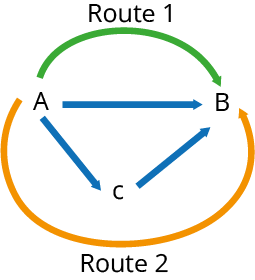• Hess' Law can be combined with the enthalpy change of production and combustion to determine the enthalpy change of a reaction.

• It's crucial to pay attention to the directions of the enthalpy change arrows in the following cycles:

• To find the enthalpy change of a reaction, using the enthalpy change of combustion: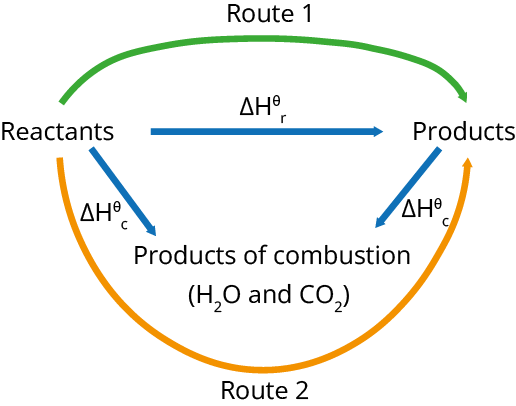• To find the enthalpy change of reaction, using the enthalpy change of formation: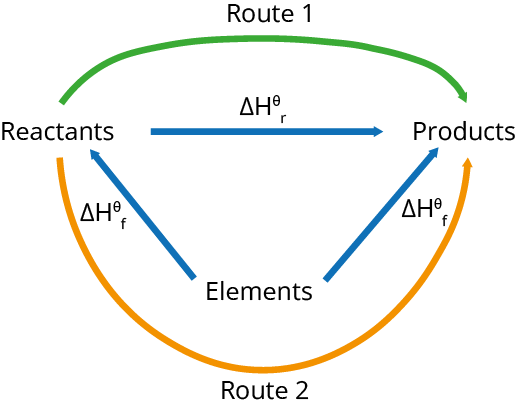### Average Bond Energies

• When all reactants and products are gaseous, bond energies can be utilised to calculate the enthalpy change of a process.

• Method 1: ΔH = total energy required to break bonds minus total energy generated during bond formation. The average bond enthalpies for each bond must be multiplied by the number of that bond included in the equation when totaling the energy released or created.

• The second method is to use an enthalpy cycle.
Example:  CO(g) + H2O(g) → CO2(g) + H2(g)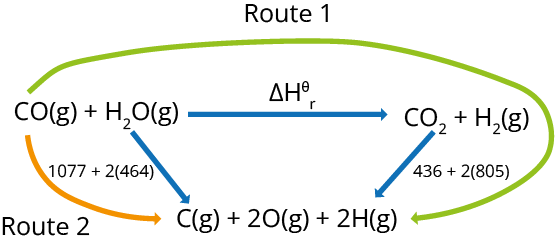Using the diagram and following:
ΔH + 436 + 2(805) = 1077 + 2 (464)

ΔH = 1077 + 2(464) - 436 - 2H = 1077 + 2(464) - 436 - 2H = 1077 + 2(464) - (805)

ΔH = -41 kJ mol-1.

### Born-Haber Cycles

• Born-Haber cycles are used to calculate lattice enthalpy. The following enthalpy changes are used in Born-Haber cycles:

• Enthalpy of formation on the lattice or enthalpy of dissociation on the lattice

• Atomisation's enthalpy shift

• Formation of enthalpy change

• The energy necessary to remove one electron from one mole of gaseous atoms in order to generate one mole of gaseous 1+ ions is known as the first ionisation energy. This is only used for metals in Born-Haber cycles.

• The energy produced when each atom in one mole of gaseous atoms receives an electron, generating one mole of gaseous 1-ions. This only applies to non-metals in Born-Haber cycles.

• A Born-Haber cycle for lithium fluoride and the computation of the lattice enthalpy (ΔHӨLE) are shown below: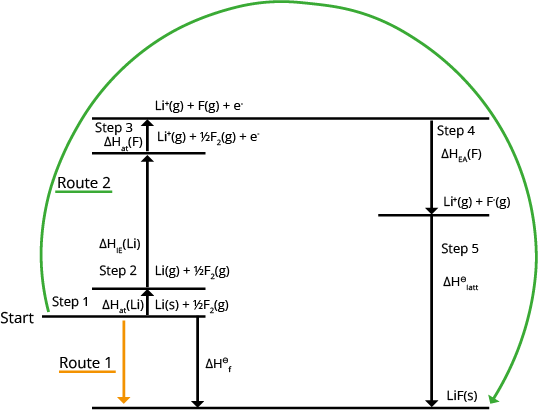### Reaction Pathway Diagrams

• The following are diagrams of exothermic and endothermic reaction pathways: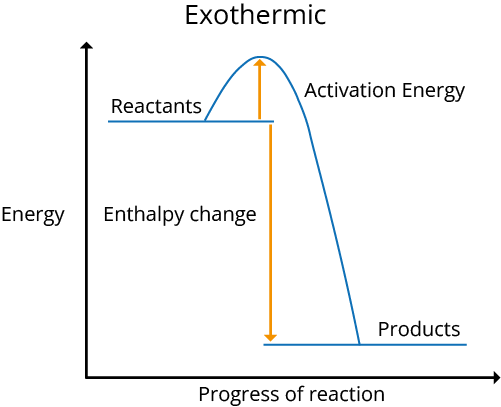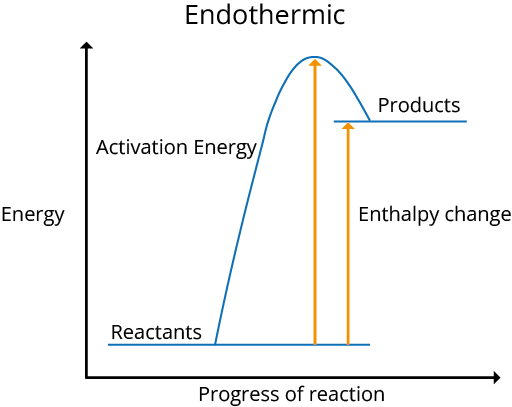### Entropy change, ΔS

• The degree of disorder in a system is measured by entropy. When there is more chaos and energy is spread out, a system becomes more stable.

• From solid to liquid to gas, entropy increases, and watery substances have higher entropy than solids. This is because the energy spreads out as the particles get more disordered as their states change.

• As gases have significantly higher entropy than liquids or solids due to their disordered mobility, the entropy change will be positive (entropy increases) if the number of gaseous moles increases throughout a process.

• Increasing the temperature may create a state shift, increasing the entropy.

• Entropy increases when the temperature rises without a change in the state because the particles have more kinetic energy and are more disordered as they move faster.

• The following equation can be used to calculate the change in entropy of a reaction:

ΔS = ΣSϴ(products) - ΣSϴ(reactants)

Where Σ means ‘the sum of’ and the standard entropies of the reactants and products have been given.

A negative result indicates a drop in entropy, whereas a positive result suggests an increase in entropy.

### Gibbs Free Energy Change, ΔG

• This equation can be used to compute Gibbs free energy change:
ΔG = ΔH - TΔS; where
ΔG - Gibbs free energy change (kJ mol-1)

ΔH - enthalpy change (kJ mol-1)

T - temperature (K)

ΔS - entropy change (kJ K-1 mol-1

• When ΔG is less than or equal to 0, a response or process is spontaneous/possible.

• If ΔG is greater than zero, the reaction is not spontaneous at the calculated temperature.

• When you have H and S, rewrite the equation as ΔH - TΔS 0 and rearrange it to find the minimal temperature at which a reaction is spontaneous.

### Spontaneity

• When ΔH is negative and ΔS is positive: TΔS is negative since H is negative and TΔS is positive in the equation. As ΔG is always negative regardless of temperature, the reaction is spontaneous at all temperatures.

• When ΔH is positive and ΔS is negative: the equation says that -TΔS is positive because ΔH is positive and TΔS is negative. ΔG is always positive and the reaction is never spontaneous because both terms are positive regardless of temperature.

• When ΔH and ΔS are both positive: Because ΔH is positive and TΔS is positive in the equation, -TΔS is negative. As the temperature rises, -TΔS becomes increasingly negative. -TΔS will overwhelm H at high temperatures, and ΔG will be less than 0, resulting in a spontaneous reaction. At low temperatures, the reaction will not be spontaneous.

• When ΔH and ΔS are both negative: TΔS is positive since ΔH is negative and TΔS is negative. At high temperatures, -TΔS becomes increasingly positive and outweighs ΔH, indicating that the reaction is not spontaneous. At low temperatures, the reaction will occur spontaneously.

### Energetics of Glycolysis

• Glycolysis is the process of breaking down glucose to produce energy. It generates two pyruvate molecules, ATP, NADH, and water.

• The process occurs in a cell's cytoplasm and does not require oxygen.

• It can be found in both aerobic and anaerobic bacteria.

• The energetics of glycolysis include the formation of two molecules of glyceraldehyde 3-phosphate from one glucose molecule in the second stage of glycolysis, and the formation of two molecules of pyruvate as end products of glycolysis.

• As a result, two molecules of glyceraldehyde 3-phosphate are used to calculate glycolysis energy.

### Solved Examples from The Chapter

Question 1: Calculate the enthalpy change of combustion for a reaction in which 0.65 g of propan-1-ol was totally combusted and 150 g of water was heated from 20.1 to 45.5oC.

Solution:

• Calculate the amount of energy that was utilised to heat the water.
Q = m x cp x ΔT,

∴ Q = 150 x 4.18 x 25.4,

∴ Q = 15925.8 J

• Calculate how many moles of alcohol were combusted.
moles of propan-1-ol = mass/ Mr (molecular weight) = 0.65 / 60 = 0.01083 mol

• ΔcH is the enthalpy change per mole that is calculated (the enthalpy change of combustion).
ΔH = Q/ no of moles

∴ ΔH = 15925.8/0.01083

∴ ΔH = 1470073 J mol-1 = 1470 kJ mol-1 to 3 sf

• Finally, add the sign to represent the energy change: if the temperature rises, the reaction is exothermic and is denoted by a negative sign, such as –1470 kJ mol-1.

Question 2: Calculate the temperature range in which this reaction can occur.
N2(g) + O2(g) → 2NO(g).

Solution:

• ∆ H = 180 kJ mol-1 ∆S = 25 J K-1 mol-1 The reaction will be feasible when ∆ G ≤0

• Make ∆G = 0 in the following equation: ∆G = ∆H - T∆S

∴ 0 = ∆H - T∆S

• So, T = ∆H / ∆S

T = 180/ (25/1000)

= 7200K

• The T must be greater than 7200K, which is a very high temperature.

### Solved Examples from Previous Year Question Papers

Question 1: When an ideal diatomic gas is heated at constant pressure the fraction of the heat energy supplied which increases the internal energy of the gas is:

(a) 2/5

(b) 3/5

(c) 3/7

(d) 5/7

Solution:

• According to the first law of thermodynamics,
ΔU = q + W

• When under constant pressure,
ΔU = qp - PΔV

qp = ΔU + PΔV

• Heat Energy Fraction that Increases Internal Energy
$= \frac{\bigtriangleup U}{q_p} \ = \frac{nC_v \bigtriangleup T}{nC_p \bigtriangleup T} \ = \frac{C_v}{C_p}$

• In the case of a diatomic gas,
Cp = 7R/2, & Cv = 5R/2.
Cv/Cp = 5/7.

• As a result, option (c) is the correct answer.

Question 2: Enthalpy change for a reaction depends upon

(a) physical state of reactants and products

(b) initial and final concentration

(c) number of steps in the reaction

(d) conditions of reaction

Solution:

• ΔH of a reaction is determined by the following conditions:

(i) Reactants and products' physical states

(ii) Concentration at the start and end

(iii) The circumstances in which the reaction took place

• As a result, option (a), (b), (d) is the correct answer.

Question 3: The equilibrium constant for the reaction, 2NOCl(g) → 2NO(g) + Cl2(g)  at 400 K is . (Given ∆Ho = 77.2 kJ mol-1; ∆So = 122 J K-1 mol-1 (log1.95 = 0.292)

(a) Kc = 1.995 x 104

(b) Kc = 1.995 x 10-3

(c) Kc = 1.995 x 10-4

(d) Kc = 1.995 x 10-4

Solution:

• ∆Go = ∆H - T∆So

• ∆Go = 77.2 x 1000 - 400 x 22

• ∆Go = 77200 - 48800

• ∆Go = 28400 Jmol

• As ∆Go = -2.303RT logKeq

• Therefore, ∆Go = 1.95 x 10-5

• As a result, option (d) is the correct answer.

### Practice Questions

Question 1: What is the temperature at which methane melts?

CH4(s) → CH4(l) ∆H = 0.94 kJmol-1, ∆S = 10.3 Jmol-1 K

(a) T = 91 K

(b) T = 87 K

(a) T = 45 K

(a) T = 95 K

Answer: (a) T = 91 K

Question 2: Calculate the enthalpy of solution of NaCl if the lattice enthalpy of production of NaCl is -771 kJmol-1 and the hydration enthalpies of sodium and chloride ions are -406 kJmol-1 and -364 kJmol-1, respectively.

(a) -1 kJ mol-1

(b) +3 kJ mol-1

(c) +1 kJ mol-1

(d) -2 kJ mol-1

### Conclusion of JEE Advanced Physical Chemistry Energetics Notes

Heat is absorbed or released in conjunction with all chemical processes. From the beginning, the intimate relationship between matter and energy has piqued people's interest and sparked discussion; it's no coincidence that fire was regarded as one of the four basic elements (together with earth, air, and water) as early as the fifth century BCE. Here we went over some of the essential ideas of energy and heat, as well as the relationship between them, in this unit.

See MoreView All Dates
JEE Advanced 2022 exam date and revised schedule have been announced by the NTA. JEE Advanced 2022 will now be conducted on 28-August-2022, and the exam registration closes on 11-August-2022. You can check the complete schedule on our site. Furthermore, you can check JEE Advanced 2022 dates for application, admit card, exam, answer key, result, counselling, etc along with other relevant information.
See More
AugustRelease of Application form
07-Aug-2022Last date to fill the form
11-Aug-202223-Aug-202228-Aug-202203-Sep-202203-Sep-202211-Sep-2022Declaration of Result
11-Sep-2022
View All DatesApplication Form
Eligibility Criteria
Reservation Policy
IIT Bombay has announced the JEE Advanced 2022 application form release date on the official website https://jeeadv.ac.in/. JEE Advanced 2022 Application Form is available on the official website for online registration. Besides JEE Advanced 2022 application form release date, learn about the application process, steps to fill the form, how to submit, exam date sheet etc online. Check our website for more details.View JEE Advanced Syllabus in Detail
It is crucial for the the engineering aspirants to know and download the JEE Advanced 2022 syllabus PDF for Maths, Physics and Chemistry. Check JEE Advanced 2022 syllabus here along with the best books and strategies to prepare for the entrance exam. Download the JEE Advanced 2022 syllabus consolidated as per the latest NTA guidelines from Vedantu for free.
See More
Paper 1
Paper 2MathsPhysics
View JEE Advanced Syllabus in Detail## JEE Advanced 2022 Study Material

View all study material for JEE Advanced
JEE Advanced 2022 Study Materials: Strengthen your fundamentals with exhaustive JEE Advanced Study Materials. It covers the entire JEE Advanced syllabus, DPP, PYP with ample objective and subjective solved problems. Free download of JEE Advanced study material for Physics, Chemistry and Maths are available on our website so that students can gear up their preparation for JEE Advanced exam 2022 with Vedantu right on time.
See More
All
Chemistry
Maths
Physics
See Allsee all
See More
PYQP
Sample Paper
2021
All Years
Paper 1
24th July 2021 - physicsEnglish  •   Shift 1views
24th July 2021 - chemistryEnglish  •   Shift 1views
30th July 2021 - mathematicsEnglish  •   Shift 1views
Paper 2
30th July 2021 - physicsEnglish  •   Shift 2views
04th August 2021 - chemistryEnglish  •   Shift 2views
04th August 2021 - mathematicsEnglish  •   Shift 2views
Paper 1
mathematicsEnglish  •   Shift NAviews
mathematicsEnglish  •   Shift NAviews
mathematicsEnglish  •   Shift NAviews
mathematicsEnglish  •   Shift NAviews
mathematicsEnglish  •   Shift NAviews
Paper 2
mathematicsEnglish  •   Shift NAviews
mathematicsEnglish  •   Shift NAviews
mathematicsEnglish  •   Shift NAviews
mathematicsEnglish  •   Shift NAviews
mathematicsEnglish  •   Shift NAviewsView all JEE Advanced Important Books
In order to prepare for JEE Advanced 2022, candidates should know the list of important books i.e. RD Sharma Solutions, NCERT Solutions, HC Verma books and RS Aggarwal Solutions. They will find the high quality readymade solutions of these books on Vedantu. These books will help them in order to prepare well for the JEE Advanced 2022 exam so that they can grab the top rank in the all India entrance exam.
See MoreMaths
NCERT Book for Class 12 MathsPhysics
NCERT Book for Class 12 Physics
See AllView all mock tests
See More
JEE Advanced 2018 Mock Test 1 - Paper 1
3 hr  • 69 questions • OBJECTIVE
JEE Advanced 2018 Mock Test 1 - Paper 2
3 hr  • 48 questions • OBJECTIVE
JEE Advanced 2018 Mock Test 2 - Paper 1
3 hr  • 69 questions • OBJECTIVE## Toppers## Master TeachersFrom IITs & other top-tier colleges with 5+ years of experienceYou can count on our specially-trained teachers to bring out the best in every student.They have taught over 4.5 crore hours to 10 lakh students in 1000+ cities in 57 countries11+ years exp

### Shreyas

Physics master teacher

#### NIT Nagpur4+ years exp

### Nidhi Sharma

Chemistry master teacher

#### Jamia Hamdard2+ years exp

### Luv Mehan

Chemistry Master Teacher

#### IISc BangaloreIIT Bombay is responsible for the release of the JEE Advanced 2022 cut off score. The qualifying percentile score might remain the same for different categories. According to the latest trends, the expected cut off mark for JEE Advanced 2022 is 50% for general category candidates, 45% for physically challenged candidates, and 40% for candidates from reserved categories. For the general category, JEE Advanced qualifying marks for 2021 ranged from 17.50%, while for OBC/SC/ST categories, they ranged from 15.75% for OBC, 8.75% for SC and 8.75% for ST category.
See MoreThe JEE Advanced 2022 result will be published by IIT Bombay on https://jeeadv.ac.in/ in the form of a scorecard. The scorecard will include the roll number, application number, candidate's personal details, and the percentile, marks, and rank of the candidate. Only those candidates who achieve the JEE Advanced cut-off will be considered qualified for the exam.
See More
Rank List
Counselling
Cutoff
JEE Advanced 2022 State Wise Rank List - The respective state authorities started releasing the JEE Advanced 2022 state merit lists for admission to Engineering course. Check JEE Advanced 2022 state-wise rank list for the year 2022 here.Want to know which Engineering colleges in India accept the JEE Advanced 2022 scores for admission to Engineering? Find the list of Engineering colleges accepting JEE Advanced scores in India, compiled by Vedantu. There are 1622 Colleges that are accepting JEE Advanced. Also find more details on Fees, Ranking, Admission, and Placement.
See More## FAQs on JEE Important Chapter - Energetics

FAQ

1. In chemistry, what is energetics?

Exothermic processes in solution release energy and raise the temperature, whereas endothermic reactions absorb energy and lower the temperature. In reactions, bonds are broken and formed. When a chemical reaction occurs, energy is transferred to or from the surroundings. This frequently results in temperature variations that may be observed with a thermometer. Exothermic and endothermic reactions are also possible. Energetics is the study of the energy changes that occur in chemical reactions, whether exothermic or endothermic.

2. Is thermodynamics and energetics the same thing?

The distinction between thermodynamics and energetics is that energetics is a field of physics that investigates the flow and transformation of energy, whereas thermodynamics is the science of energy transformations.

3. What is the fundamental principle of chemistry and energetics?

By releasing or absorbing heat, all chemical reactions exchange energy with their surroundings. Energy is exchanged as pressure-volume work in reactions that produce or consume gases. Exothermic means that a chemical process produces heat. It is endothermic when a chemical reaction absorbs heat.Last date for submitting JEE Advanced application form
12 Sep 202230 days remaining28 Sep 202245 days remaining## Notice board

News
BlogJEE Advanced 2022 Registration Ends Today
10 Aug 2022JEE Main 2022 Cutoff Released: Check Details Here
09 Aug 2022JEE Advanced 2022: Registration Window Open for Foreign Nationals
03 Aug 2022JEE Advanced 2022: Check Registration, Exam Pattern & Dates Details
29 Jul 2022JEE Main 2022 Results: Meet Vedans Who Scored 99.9 Percentile and Above
28 Jul 2022Trending pages